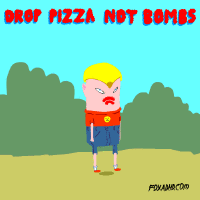# Pizza War 1Vaibhav gives a challenge to Kalash. He says that describe a motion such that the velocity vector at some instant of time makes an angle of $45^{\circ}$ with the acceleration vector. Kalash then swiftly answers that $\vec{r} = at\hat{i} + at\left(1 - \dfrac{t}{10}\right)\hat{j}$.

Kalash then asks Vaibhav at what instant of time will this occur for the motion described above. Vaibhav is confused. Can you help him out?

×

Problem Loading...

Note Loading...

Set Loading...